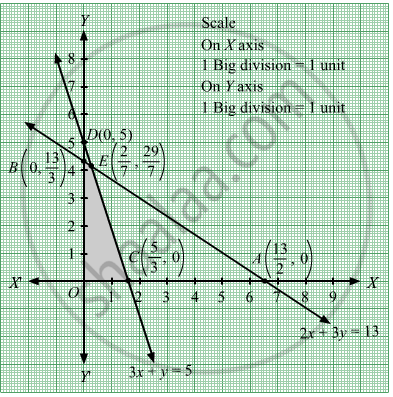# Maximize Z = 9x + 3y Subject to 2 X + 3 Y ≤ 13 3 X + Y ≤ 5 X , Y ≥ 0 - Mathematics

Sum

Maximize Z = 9x + 3y
Subject to

$2x + 3y \leq 13$

$3x + y \leq 5$

$x, y \geq 0$

#### Solution

First, we will convert the given inequations into equations, we obtain the following equations:
2x + 3y = 13, 3x +y = 5, x = 0 and y = 0
Region represented by 2x + 3y ≤ 13 :
The line 2x + 3y = 13 meets the coordinate axes at $A\left( \frac{13}{2}, 0 \right)$ and  $B\left( 0, \frac{13}{3} \right)$  respectively. By joining these points we obtain the line 2x + 3y = 13.

Clearly (0,0) satisfies the inequation 2x + 3y ≤ 13. So,the region containing the origin represents the solution set of the inequation 2x + 3y ≤ 13.
Region represented by 3x + y ≤ 5:
The line 5x + 2y = 10 meets the coordinate axes at
$C\left( \frac{5}{3}, 0 \right)$ and D(0, 5) respectively. By joining these points we obtain the line 3x y = 5.
Clearly (0,0) satisfies the inequation 3x + y ≤ 5. So,the region containing the origin represents the solution set of the inequation 3x + y ≤ 5.
Region represented by x ≥ 0 and y ≥ 0:

Since, every point in the first quadrant satisfies these inequations. So, the first quadrant is the region represented by the inequations x ≥ 0, and ≥ 0.
The feasible region determined by the system of constraints, 2x + 3y ≤ 13, 3x + y ≤ 5, x ≥ 0, and y ≥ 0, are as follows.The corner points of the feasible region are O(0, 0),

$C\left( \frac{5}{3}, 0 \right)$ $E\left( \frac{2}{7}, \frac{29}{7} \right)$ and  $B\left( 0, \frac{13}{3} \right)$

The values of Z at these corner points are as follows.

We see that the maximum value of the objective function Z is 15 which is at C

 Corner point Z = 9x + 3y O(0, 0) 9 × 0 + 3 × 0 = 0 $C\left( \frac{5}{3}, 0 \right)$ 9 × $\frac{5}{3}$ + 3 × 0 =15 $E\left( \frac{2}{7}, \frac{29}{7} \right)$ 9 × $\frac{2}{7}$ +3 × $\frac{29}{7}$ = 15 $B\left( 0, \frac{13}{3} \right)$ 9 × 0 +3 × $\frac{13}{3}$ = 113
$\left( \frac{5}{3}, 0 \right)$ and  $E\left( \frac{2}{7}, \frac{29}{7} \right)$  Thus, the optimal value of Z is 15.
Concept: Graphical Method of Solving Linear Programming Problems
Is there an error in this question or solution?

#### APPEARS IN

RD Sharma Class 12 Maths
Chapter 30 Linear programming
Exercise 30.2 | Q 2 | Page 32